﻿ Improving Accuracy of Educational Research Conclusions by Using Lisrel

### Improving Accuracy of Educational Research Conclusions by Using Lisrel

Awaluddin TjallaOPEN ACCESSPEER-REVIEWED

## Improving Accuracy of Educational Research Conclusions by Using Lisrel

Awaluddin TjallaDepartment of Guidance and Counseling, State University of Jakarta, Kampus UNJ, Jl. Rawamangun Muka, Rawamangun, Jakarta

### Abstract

The purpose of this paper are (1) to propose applying a technique of data analysis of educational variables using LISREL, (2) to construct variables including in the research model. The other benefit of this technique compared to the conventional analysis model are: (1) faulty estimate on variables relation caused by measurement error can be corrected and (2) statistical test on whether or not a theoritical model describing relation structure between variables can be carried out. The other benefit is that fit between theoritical model and data can be tested. The use of LISREL technique to analyze variable data for education is a must. Sharpness and accuracy in predicting the variables which are considered to have influence on variables can be obtained. On the contrary, measurement error which takes place from relation between research variables can be explained. Accordingly, this analysis technique is considered “comprehensive” to improve accuracy of conclusion generalization in the field of educational research which currently undergoes more complex problem.

### At a glance: Figures

1
Prev Next

• Tjalla, Awaluddin. "Improving Accuracy of Educational Research Conclusions by Using Lisrel." American Journal of Educational Research 3.5 (2015): 619-623.
• Tjalla, A. (2015). Improving Accuracy of Educational Research Conclusions by Using Lisrel. American Journal of Educational Research, 3(5), 619-623.
• Tjalla, Awaluddin. "Improving Accuracy of Educational Research Conclusions by Using Lisrel." American Journal of Educational Research 3, no. 5 (2015): 619-623.

 Import into BibTeX Import into EndNote Import into RefMan Import into RefWorks

### 1. Introduction

Globalization which is characterized by advancement in technology has resulted in problems on human life interaction, including those in educational field. In the perspective of research methodology, augmenting problems in educational field either quantitatively or qualitatively require settlement with correct technique of data analysis. This is aimed at obtaining objective result of data analysis and can be generalized accurately. Data analysis in educational field is not as easy as that in the other fields, such as : industry, engineering, agriculture, economics, etc., in which their required statistical analysis can easily be fulfilled. Interpretation made does not bear high risk compared to the more complex data of educational variables. Therefore, correct technique of statistical analysis is required.

Pophamand Sirotnik (1973) mentioned two main objectives of statistical use in a bid to analyze variables data in the educational field, they are :

1. Statistical techniques to describe data (descriptive satistics). This statistics is used to infer numerical data, such as : test scores, age and educational year.

2. Statistics used by researchers to describe better inference against a phenomenom observed at sample and then generalized conclusion of population is taken. This analysis technique points to relation between variables. In such case, educational researchers try to describe relation between such variables as students’ IQ, achievement and attitude to learning program at school.

With regard to the second objective of using statistics (inferensial statistics),the commonly used technique of statistical analysis in the educational field is regression analysis by applying production function approach (Draper and Smith, 1981) . However, such model constitutes underlying weaknesses in terms of : (1) concept, (2) result measurement, and (3) some biased sources which are the characteristics in the educational field. Accordingly, by applying regression with Ordinary Least Squares (OLS) approach, such disturbances need serious attention and required assumption can be met. This is because assumption break may cause inccorrect and misleading conclusion generalization due to possible misinterpretation (Suriasumantri, 2000) .

### 2. Analysis

Path analysis is commonly applied as well in analyzing educational variables data irrespective of its weaknesses. The following are the weaknesses (Mueller, 1996) : (1) When assumption of series of variables events (one variable cannot precede the other and vice versa) is not met, then path analysis cannot be applied; (2) It is always assumed that error outside the system has no correlation between one and another; (3) Difficulty in determining unknown parameters of the available data; (4) Direction of cause based relation cannot be determined by analysis result but dependent upon the concept developed by researchers. In case of any faulty concept, then conclusion and interpretation will be faulty as well; and (5) Principally, path analysis is the application of equation of the Two Stage LeastSquares (TSLS) which bases as well on the Ordinary Least Squares (OLS). Accordingly, when the required assumption by OLS is not fulfilled, then the result will be biased too.

In comparison with regression analysis, path analysis possesses some excellence (James, Mulaik, and Brett, 1982) . This is because we can make influence decomposition of the variables, know how huge the direct and indirect influence as well as that which does not belong to the cause based one. Besides, we can arrange an interesting model on cause based relation between free and tied variables. However, difficulty frequently arises when it comes to making or arranging series of events of such variables.

Figure 1. LISREL model consisting of measurement and structural components

At the measurement model analysis, latent variable cannot be directly measured (as a factor or construct, such as chidren’s spacial ability). Loadingat each observed variablefor one factor shows correlation between the researched construct and at the common variancewith other variable identified as latent variable (Kim and Mueller, 1978) .

Equation model used for component of measurement equation is as follows :

1. For measurement equation model x (exogenous model) is :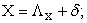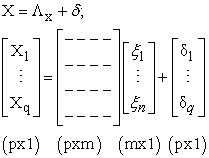2. For measurement equation model y (endogenous variable) is as follows :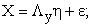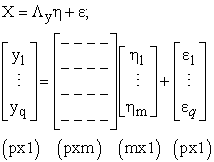Where: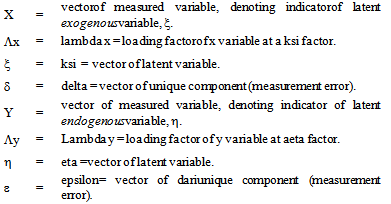Both of the above measurement equations (for x and y) are the same, showing relation between measured variables x and y with their latent variables (factor to be measured) ξand η.

Structural equation model points to latent variables analysis (Loehlin, 1992) . This term is used as structural equation modeldetermines relation between latent variables. Notation used for structural equation modelis as follows: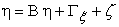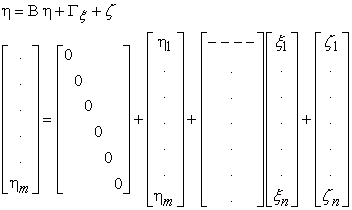Where: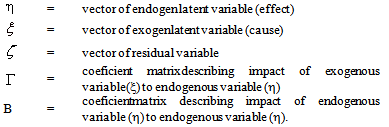Basically, structural equation model belongs to equation in the form of matrix of parameters, exogen variables, endogen variables and residual. Detail or element of each matrix is much dependent upon the number of latent variables used and line of cause based relation described. Hence, application of the structural equation highly depends on cause based relation model designed to describe a phenomenon.

Statistically, there are 7 (seven) assumptions to be fulfilled (Mueller, 1996) prior to conducting a test to structural model, they are :

1. Exogenousand endogenous latent variablespossess ‾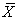= 0 ; [ E ( ξ ) = E ( η ) = 0 ] ;

2. Structural relation of exogenousto endogenous latent variables is linear;

3. Error for ζ at structural equation model: (a) having average same as zero [ E ( ζ ) = 0 ]; (b) independent, and (c) has no correlation with latent variable [ E (ξζ) = E (ξζ) = 0 ];

4. Matrix (I – B) is not single, a matrix which cannot be inversed.

5. Average of observed variable of the exogeneous and endogeneous variables is 0, that is E (X) = E (Y) = 0.

6. Relation between indicatorsof exogeneousand endogeneous variables and latent variables (either exogeneousor endogeneous) is linear.

7. Error measurement forδand εin measurement equations x and y: (a) possesses average of 0 [E (δ) = E (ε) = 0]; (b) independent; (c) exogeneousand endogeneous latent variables are not correlated [E (ξδ ) = E (δξ ) = 0; E (ηδ ) = E (δη ) = 0 ; E (ηε ) = E (εη ) = 0; and E ( ξε ) = E (εξ); and (d) do not have any correlation between measurement error [E (εδ ) = E (δε) = 0 ].

In LISREL model there are two stages which are related one another, they are : (1) to test the model truth by seeing whether there exists significant difference between model and data and (2) in case of any accord between model and data, a test of hyphothesis on structural relation in such model can be carried out (Hair et.al, 1998) . To test the fit between theoritical model proposed with data a goodness-of-fit test can be applied. This test describes how far the arranged theoritical model is supported by data. Some fit test parameters between model and data cover : Chi-square (χ2); Indeks Goodness-of-fit (GFI); Indeks Adjusted Goodness-of-Fit (AGFI) and root of residual variance residuor RMR (Byrne, 1998) . In this case datameans covariant matrixes between measured variables, they are x and y, and theoritical model is covariant matrixes between x and ywhich are expected to be secured if the model is correct. Calculation formula of the expected matrixes can be made as each x and y denotes function of latent variables together with their parameters.

In order to obtain a model which is in accord with data, chi-square (χ2) achieved has to possess probability ≥ 0.05 (Hayduk, 1988) . If zero hypothesiswhich states that there is no distinction between model with data cannot be rejected (not significant), it means there is no difference between model and data. In other words, the proposed theoritical model fits to describe data. In furtherance, the fit test between model and data can also be carried out by seeing value magnitude of GFI, AGFI and RMR. The more huge the valueof GFI and AGFI obtained (ranging from 0 – 1) and the smaller the value of RMR, it means that the proposed model is good. However, a model test by an index other than chi-square2) is non-probabilistic (cannot be used to determine significance level of the existing difference).

The research is conducted by Maruyama and Miller (1979) , in USA by applying LISREL to analyze data from Lewis dan St. John (1974)on influence of class mixingto learningachievement. Reference used is that norms and values possessed by white-skinned students from middle class and achievement oriented will be accepted and internalized by black-skinned students if the white-skinned students are frindly to them. The proposed LISREL model is exhibited at Figure 2.

Remarks :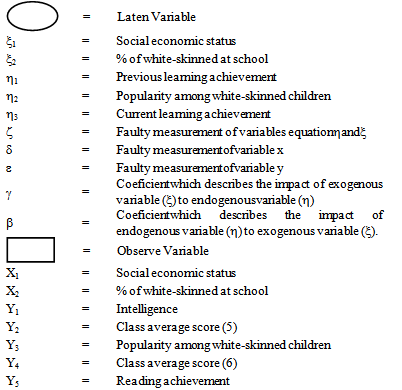In furtherance, by applying LISREL as an analysis tool proposed by Maruyama and Miller , it is obtained that the value χ2is 4,88 and not significant at the significance level of 5 % ( p >α ) with sample of 154 students. This result shows that the proposed LISREL model is fitted with the data.The coeficient magnitude between exogeneous and endogeneousvariables as well as between endogeneousand other endogeneousvariables is seen at Table 1.

#### Table 1. Result of Analysis of Stuctural Equation Model on Direct Impact of Exogen Variable to Endogen Variableas well as Endogen Variable to Other Endogen VariableDownload asPowerPoint Slide

Veiw figureView current table in a new window

Based on the analysis result, it is shown that parametersγ11, γ12 , β21and β31are significant, while parametersγ22and β32are not significant. The criteria to determine whether it is or not significantis through estimate value from value t ≥ 1.96 (Byrne, 1998) .

1. Family SES (ξ1) has influence on the previous learning achievement (η1);

2. Persent age (%) of white-skinned at school (ξ2) has influence on the previous learning achievement (η1) and does not influence the popularity of the white-skinned (η2);

3. The previous learning achievement (η1) has influence on the current learning achievement (η3);

4. Popularity among white-skinned (η2) has no influence on the current learning achievement;

5. There is no significant relation between family SES (ξ1) and percentage (%) of white-skinned at school (ξ2).

Figure 3. Result of model analysis of structural equation on influence of class mixing to learning achievement

Based on the hypothesisproposed by Maruyama and Miller on transmission of cultural value, if correct, then acceptance of black-skinned children by white-skinned children has influence on learning achievement of black-skinned childrenpositively and substantially. However, with insignificant coeficient value at β32 (.02), showing that the hypothesis of cultural transmission is not correct and that the previous learning achievement influences the current one and popularity or acceptance by the white-skinned children.

### 3. Discussion

To overcome the weaknesses in applying analysis techniques, it is proposed to use a model which is currently developed and made popular in a bid to analyze educational variables data known as LISREL (Linear Structural Relationships). This analysis technique is also commonly called “analysis of covariance structures, the moments structure model, and latent variable equation systems in structured linear models” (Long, 1983) .

This analysis technique belongs to culmination of contemporary methodology (Kerlinger, 1986) . This technique allows inclusion of latent construct variables, in which it cannot be included in regression model and path analysis. The excellence of this model compared to the conventional ones such as regression and path analyses are: (1) estimate error on relation between variables due to measurement error can be well corrected and (2) statistical test on whether a theoritical model can be accepted or not which describes relation structure between variables can be carried out.

LISREL analysis techniques to examine the fit between the theoretical model with data. In case of no significant distinction between covariant matrix expected based on model or theory and that obtained from data, it can be interpreted as proof of the model’s truth. When a theoritical model supported by data (empirical evidence)has been found, the meaningfulness of each coeficient which is based on cause relation that exists in the model can be tested as well (JoreskogdanSorbom, 1984) .

In LISREL technique, there are two groups of equations (Schumacker and Lomax, 1996) , they are measurement and structural equations. The former belongs to factor analysisused for explorative analysis and aimed at stipulating measured variables which can be made as a good indicator for latent variables. In other words, measurement model is made to estimate factor loadingfor each variable which is theoritically stipulated as an indicator of latent variables or factors. The latter is used to describe cause based relation between latent variables, either between causing variables (exogenous) or between effect variables (endogenous)as well as being used to describe the magnitudeof explained and unexplained variances.

### 4. Conclusion

The use of LISREL in analyzing educational variables is a must as today this analysis model belongs to the most comprehensive one for analysis of behavioral variables. Sharpness and accuracy in predicting variables are considered to have influence on other variables can be executed. In addition, wrong measurement arising out of relation between variables can be explained. Accordingly, this analysis thecnique is considered “comprehensive” to improve accuracy of conclusion generalization in the field of educational research which currently undergoes more complex problem.

In order to apply LISREL program, supporting knowledge and skill are required as to facilitate us in using this technique. This includes knowledge of mathematics andcomputer skill besides theoritical mastery to the research model proposed by the researchers.

### References

  Britt, David W, A Conceptual introduction to modeling: Qualitative and quantitative perspective. Lawrence Erlbaum Associates, New Jersey, 1997.In article  Byrne, Barbara M, Structural equation modeling with LISREL, PRELIS, and SIMPLIS: Basic concepts, applications, and programming, Lawrence Erlbaum Associates, New Jersey,1998.In article  Draper, N. R., and Smith, H,.Applied regression analysis (Second edition), John Wiley & Sons, Inc., New York, 1981.In article  Hair, Joseph, H., Anderson, Rolph, E., Tatham, Ronald L., and Black, William C, Multivariate data analysis (Fifth edition), Prentice-Hall International, Inc., New Jersey, 1998.In article  Hayduk, Leslie A. Structural equation modeling with LISREL. The Johns Hopkins University Press, Maryland, 1988.In article  James, Lawrence R., Mulaik, Stanley A., and Brett, Jeanne M, Causal analysis: Assumptions, models, and data, SAGE Publications, Inc., California, 1982.In article  Joreskog, Karl G., and Dag Sorbom. Advances in factor analysis and structural equation models,Abt Books, Massachusetts, 1979.In article  Joreskog, Karl G., and Dag Sorbom, LISREL VI: Analysis of linier structural relationships by the method of maximum likelihood (Third Edition), University of Uppsala, Sweden, 1984.In article  Joreskog, Karl G., danSorbom, Dag, LISREL 8; User’s reference guide. Scientific Software International, Inc., Chicago, 1996.In article  Joreskog, Karl G., Sorbom, Dag., Du toit Stephen, and Mathilda, LISREL 8: New statistical features. Scientific Software International, Inc., Chicago, 2000.In article  Keith, Timothy Z. Multiple regression and beyond. Pearson Education, Inc., Boston, 2006.In article  Kerlinger, F.N..Foundations of behavioral research (Third edition), Holt, Rinehart and Winston Inc., Florida, 1986.In article  Kim, J., and Mueller, C.W., Introduction to factor analysis: What it is and how to do it, SAGE Publications, Inc., Beverly Hills, CA, 1978.In article  Lewis, R., and St. John, S., “Contribution of cross-racial friendship to minority group achievement in desegregated classrooms,” Sociometry, 37, 79-91, 1974.In article CrossRef  Loehlin, John C. Latent variable models: An introduction to factor, path, andstructural analysis, Lawrence Erlbaum Associates Publishers, New Jersey, 1992.In article  Long, Scott J..Covariance sructure models: An introduction to LISREL. SAGE Publications Inc., California, 1983.In article  Maruyama, G., dan Miller, N., Reexamination of normative influences processes in desegregated classrooms. American Educational Research Journal,16, 273-283,1979.In article CrossRef  Mueller, Ralph O. Basic principles of structural equation modeling.: An introduction to LISREL and EQS. Springe-Verlag New York, Inc., New York, 1996.In article CrossRef  Popham, James W., danSirotnik, Kenneth A. Educational statistics use and interpretation (Second edition), Harper & Row, Publishers, Inc, New York, 1973.In article  Reisinger, Yvette.,dan Turner W, Lindsey. Cross-cultural behaviour in tourism: Concepts and analysis.Elseiver Butterworth-Heinemann, Burlington MA, 2003.In article  Schumacker, Randall E., dan Lomax, Richard G., A Beginner’s guide to structural equation modeling, Lawrence Erlbaum Associates, Publishers, New Jersey, 1996.In article  Suriasumantri, Jujun S., Philosophy of science: A popular introduction, PustakaSinarHarapan, Jakarta, 2000.In article